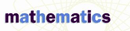# Central Tendency, Measures Of - Research Article from Macmillan Science Library: Mathematics

This encyclopedia article consists of approximately 4 pages of information about Central Tendency, Measures Of.
 This section contains 931 words (approx. 4 pages at 300 words per page) View a FREE sampleAny set of data consisting of numbers has important measurements that can be used to describe the entire set. One of these measurements is a single number that is considered the most representative of the set. Such a number is called "a measure of central tendency." Three such measures are commonly used, and each has its own advantages. These measures are known as the mean, median, and mode.

For numerical data, the "mean" (sometimes called the arithmetic average or arithmetic mean because there are other measures in mathematics also called the mean) is the sum of the values in a data set divided by the total number of values. The "median" of a set of data is the middle piece of the data after the values have been sorted from smallest to largest (or largest to smallest). For a set of data...

 This section contains 931 words (approx. 4 pages at 300 words per page) View a FREE sample